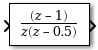# Discrete Zero-Pole

Model system defined by zeros and poles of discrete transfer function

• Library:
• Simulink / Discrete

•## Description

The Discrete Zero-Pole block models a discrete system defined by the zeros, poles, and gain of a z-domain transfer function. This block assumes that the transfer function has the following form:

`$H\left(z\right)=K\frac{Z\left(z\right)}{P\left(z\right)}=K\frac{\left(z-{Z}_{1}\right)\left(z-{Z}_{2}\right)...\left(z-{Z}_{m}\right)}{\left(z-{P}_{1}\right)\left(z-{P}_{2}\right)...\left(z-{P}_{n}\right)},$`

where Z represents the zeros vector, P the poles vector, and K the gain. The number of poles must be greater than or equal to the number of zeros (n ≥ m). If the poles and zeros are complex, they must be complex conjugate pairs.

The block displays the transfer function depending on how the parameters are specified. See Zero-Pole for more information.

### Modeling a Single-Output System

For a single-output system, the input and the output of the block are scalar time-domain signals. To model this system:

1. Enter a vector for the zeros of the transfer function in the Zeros field.

2. Enter a vector for the poles of the transfer function in the Poles field.

3. Enter a 1-by-1 vector for the gain of the transfer function in the Gain field.

### Modeling a Multiple-Output System

For a multiple-output system, the block input is a scalar and the output is a vector, where each element is an output of the system. To model this system:

1. Enter a matrix of zeros in the Zeros field.

Each column of this matrix contains the zeros of a transfer function that relates the system input to one of the outputs.

2. Enter a vector for the poles common to all transfer functions of the system in the Poles field.

3. Enter a vector of gains in the Gain field.

Each element is the gain of the corresponding transfer function in Zeros.

Each element of the output vector corresponds to a column in Zeros.

## Ports

### Input

expand all

Input signal specified as a real-valued scalar.

Data Types: `single` | `double`

### Output

expand all

Model of system as defined by zeros, poles, and gain of discrete transfer function. The width of the output is equal to the number of columns in the Zeros matrix, or one if Zeros is a vector.

Data Types: `single` | `double`

## Parameters

expand all

### Main

Specify the vector or matrix of zeros. The number of zeros must be less than or equal to the number of poles. If the poles and zeros are complex, they must be complex conjugate pairs.

• For a single-output system, enter a vector for the zeros of the transfer function.

• For a multiple-output system, enter a matrix. Each column of the matrix contains the zeros of a transfer function that relates the system input to one of the outputs.

#### Programmatic Use

 Block Parameter: `Zeros` Type: character vector Values: vector Default: `''`

Specify the vector of poles. The number of poles must be greater than or equal to the number of zeros. If the poles and zeros are complex, they must be complex conjugate pairs.

• For a single-output system, enter a vector for the poles of the transfer function.

• For a multiple-output system, enter a vector for the poles common to all transfer functions of the system.

#### Programmatic Use

 Block Parameter: `Poles` Type: character vector Values: vector Default: `'[0 0.5]'`

Specify vector of gain values.

• For a single-output system, enter a scalar or 1-by-1 vector for the gain of the transfer function.

• For a multiple-output system, enter a vector of gains. Each element is the gain of the corresponding transfer function in Zeros.

#### Programmatic Use

 Block Parameter: `Gain` Type: character vector Values: scalar | vector Default: `'1'`

Specify the time interval between samples. For more information, see Specifying Sample Time.

#### Programmatic Use

 Block Parameter: `SampleTime` Type: character vector Values: scalar | vector Default: `'-1'`

### State Attributes

Use this parameter to assign a unique name to the block state. The default is `' '`. When this field is blank, no name is assigned. When using this parameter, remember these considerations:

• A valid identifier starts with an alphabetic or underscore character, followed by alphanumeric or underscore characters.

• The state name applies only to the selected block.

This parameter enables State name must resolve to Simulink signal object when you click Apply.

For more information, see C Code Generation Configuration for Model Interface Elements (Simulink Coder).

#### Programmatic Use

 Block Parameter: `StateName` Type: character vector Values: unique name Default: `''`

Select this check box to require that the state name resolves to a Simulink® signal object.

#### Dependencies

To enable this parameter, specify a value for State name. This parameter appears only if you set the model configuration parameter Signal resolution to a value other than `None`.

#### Programmatic Use

 Block Parameter: `StateMustResolveToSignalObject` Type: character vector Values: `'off' | 'on'` Default: `'off'`

## Block Characteristics

 Data Types `double` | `single` Direct Feedthrough `yes` Multidimensional Signals `no` Variable-Size Signals `no` Zero-Crossing Detection `no`

## Version History

Introduced before R2006a